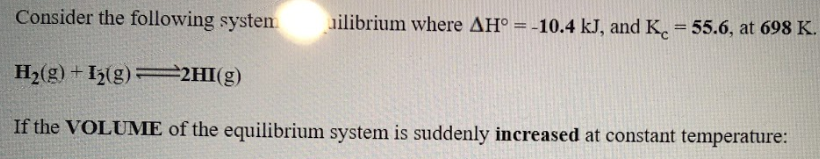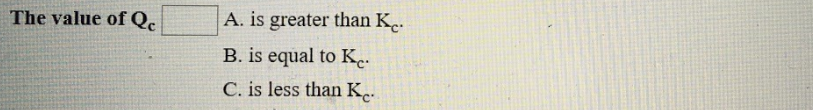# Consider the following system at equilibrium where ΔH° = -10.4 kJ, and Kc = 55.6, at 698 K. H2(g) ⇌ I2(g) + 2HI(g) If the VOLUME of the equilibrium system is suddenly increased at constant temperature: The value of Qc a) is greater than Kc. v) is equal to Kc c) is less than Kc